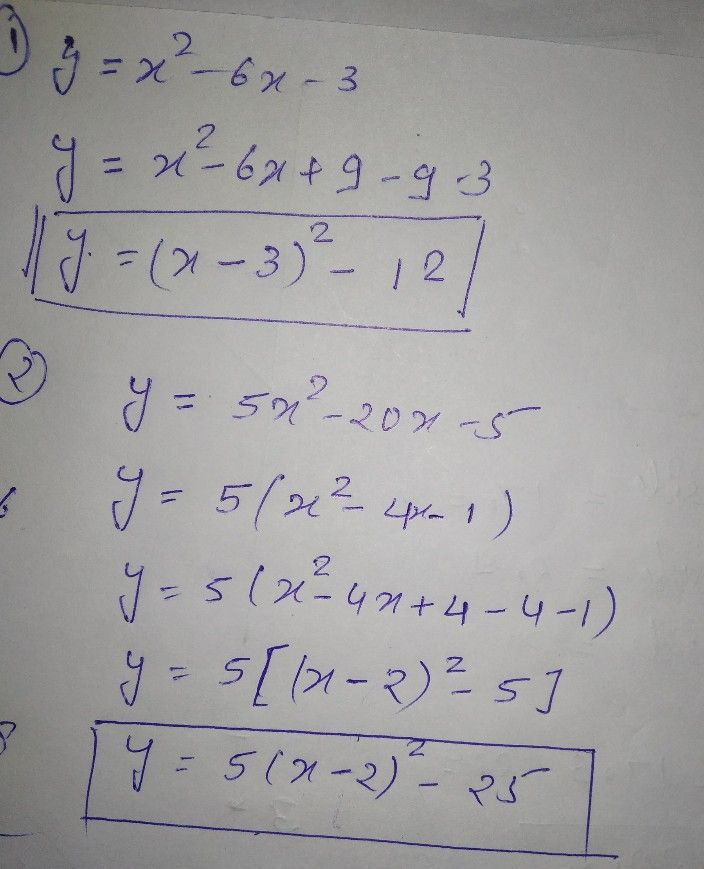Symbol
Problem$13$ Learning Task $3.$ Transform the quadratic function defined $byy=ax^{2+bx+}$ c into the form $y=a\left(x-h\right)2+k$ $1.$ $y=x^{2-6x-3}$ $2$ $y=5x^{2}-20x-5$ $4$ Learning Task $4$ Suppose that $y$ you will put a square carpet on a floor, you need a carpet with an area of $\left(x^{2-8x+k}\right)$ square meters. What will be the value of "k" so that it will make it a perfect square? $Carpe1$ $\left(62=853513\right)$ sqm.
7th-9th grade
Other
Search count: 106
SolutionQanda teacher - Tarun